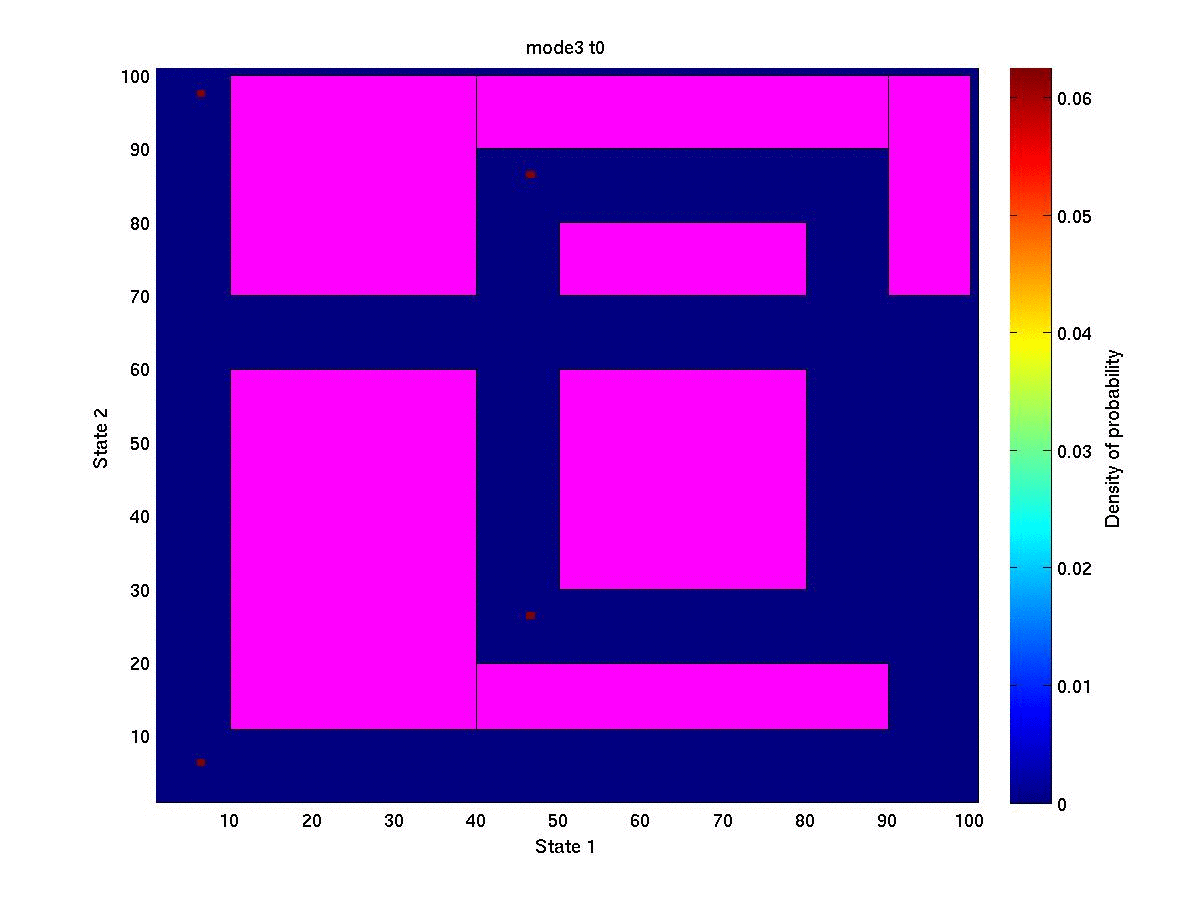# MouseS & maze: Extended Edition

To illustrate the process law on a stochastic switched system, we consider the maze & mouse problem. We add some noise in the dynamics by setting the simple volatility$Latex formula$

As initial state distribution we take two Dirac located at (0.05,0.95) and (0.05,0.05).
The corresponding file processLaw/initialDistribution_modei.t0 is given below.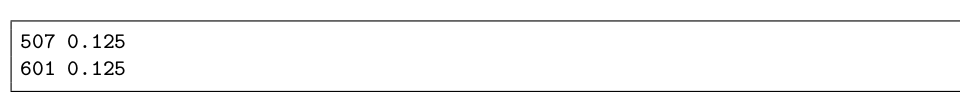Note that we need to give the initial distribution for each discrete mode. We take the same values for all modes i={0,1,2,3}. The sum of all probabilities is 0.25 for each of the four files, with a total of 1.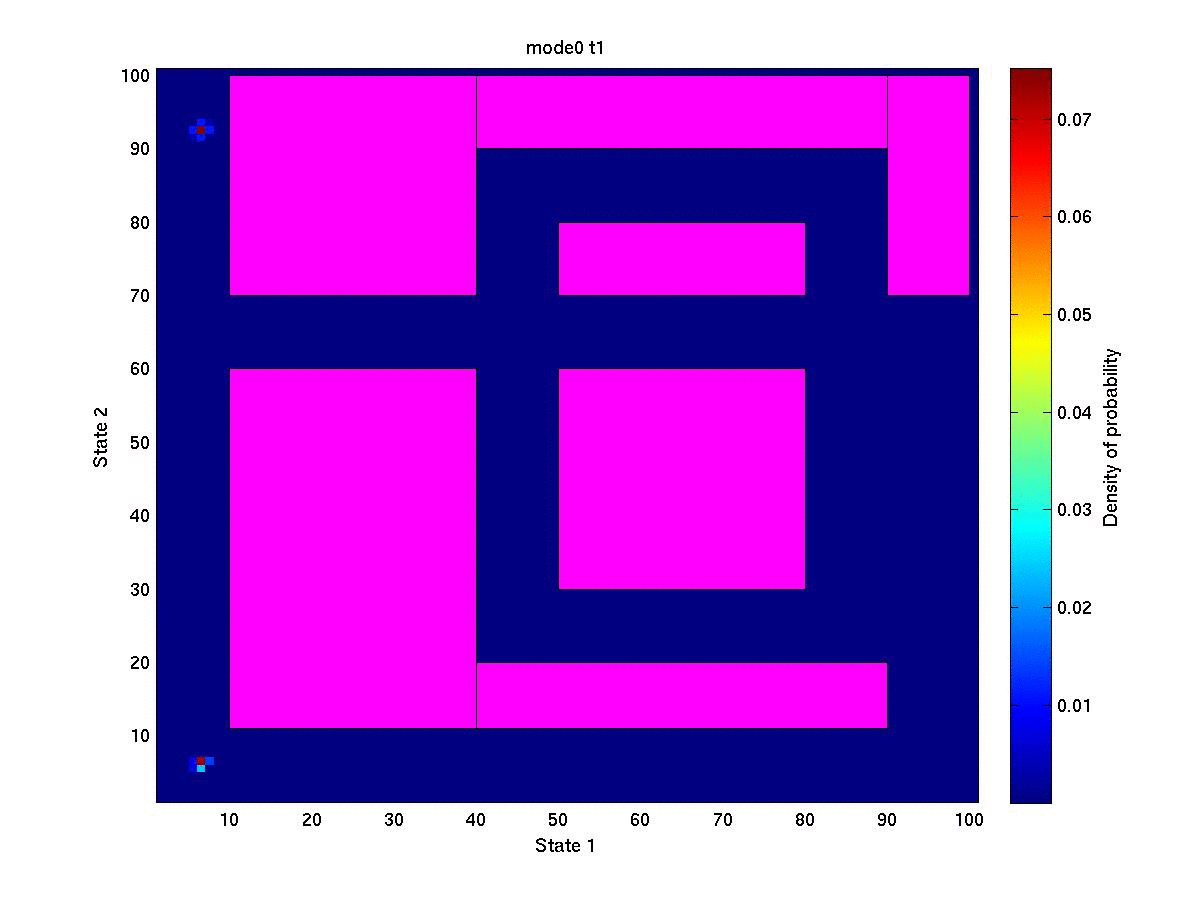Now we populate the maze a bit more ans set the initial state distribution as four Dirac located at (0.05,0.95), (0.05,0.05), (0.45,0.25) and (0.45,0.85). The corresponding file is given below. Once again the total sum of probabilities over all modes is equal to 1.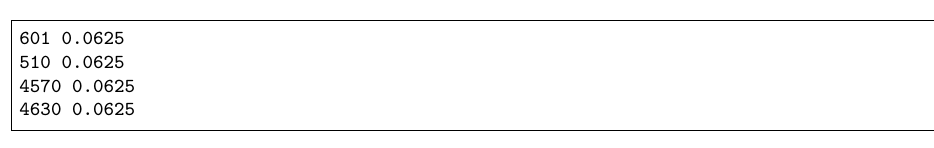We see the four groups of mouses race to the exit. Cute, aren’t they ?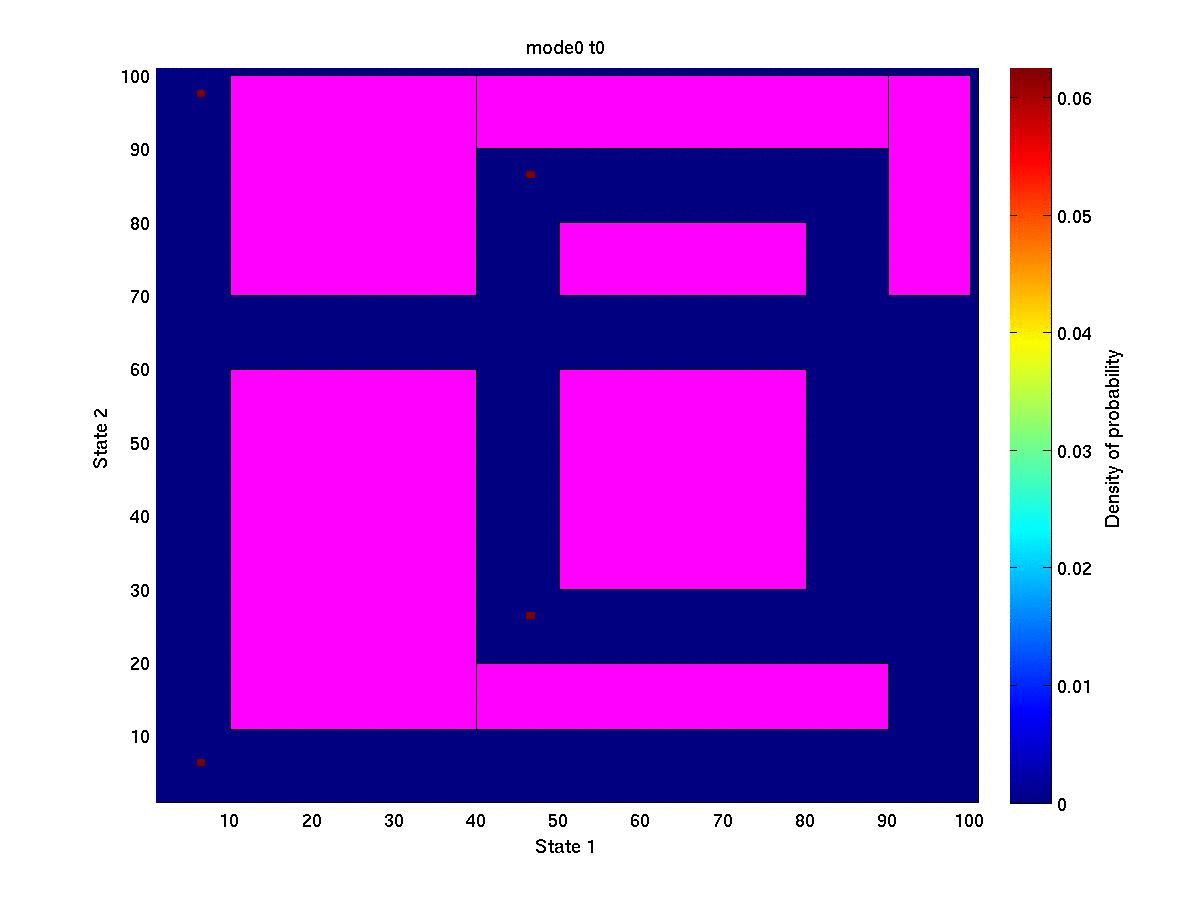Finally, we move the exit of the maze to (0.05,0.65). The four packs go for it.